# For loop in Python

### For loop in python:

For loop in python is used to traverse through a sequence of lists, tuples, or any iterable objects.

For loop in python works quite similar to an iterator that is  found in other programming languages like Java.., Where we usually do not use indexes to get items from the iterable object.

Syntax: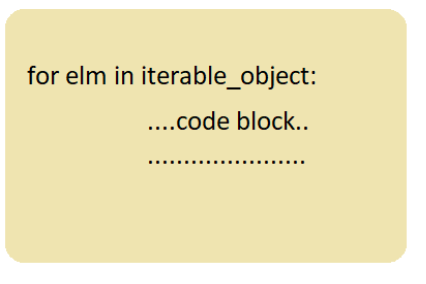In each iteration, elm takes the item from the sequence/iterable_object

For example, if the loop is running on a list = [10,22,31], in the first iteration elm will have 10, and in the 2nd iteration, elm will have 22 and in the last iteration elm will have 31

Loop will run till the last element in the sequence.

Flow chart for better understanding: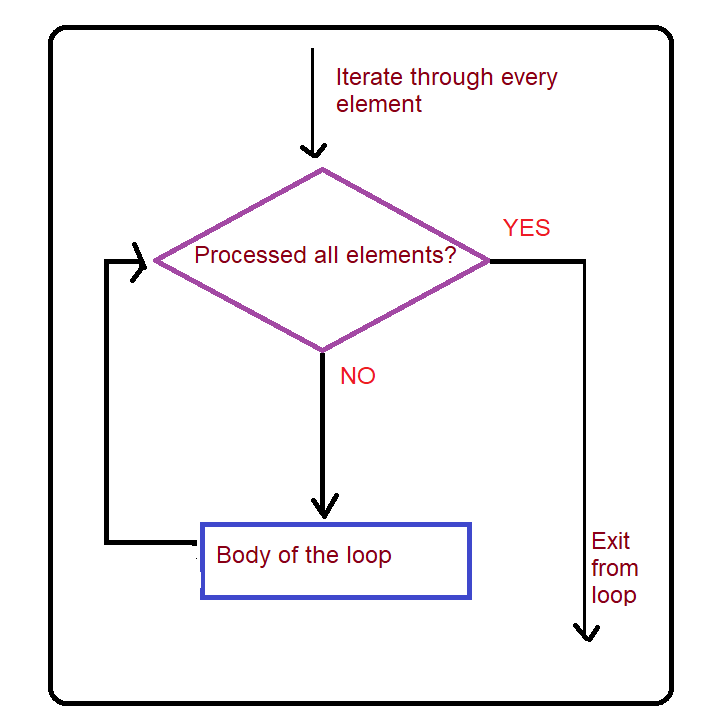Example 1 :

Program to get even numbers from  the list using for loop

Code:

## Python Code

``````myList = [3,6,2,9,24]

for elm in myList:
#check even or not
if(elm%2 == 0):
print(elm)
``````

Output:

6
2
24

#### How does the above code work?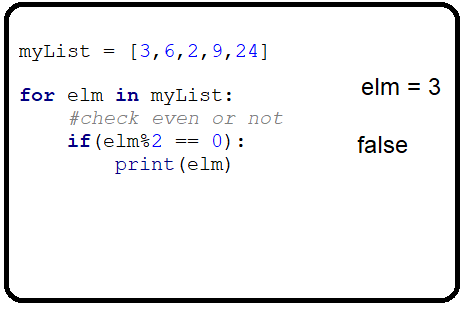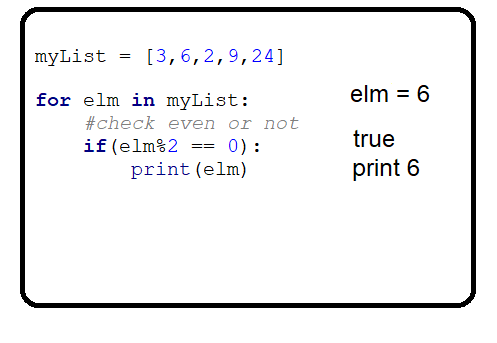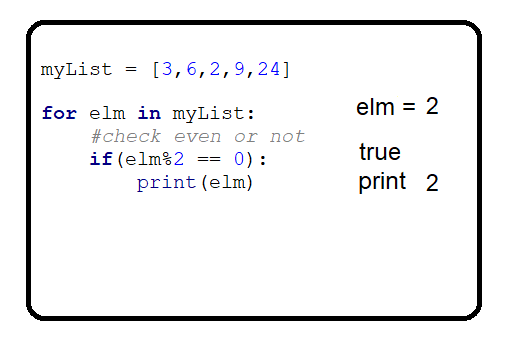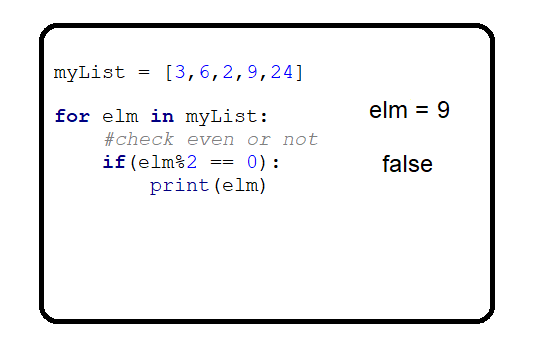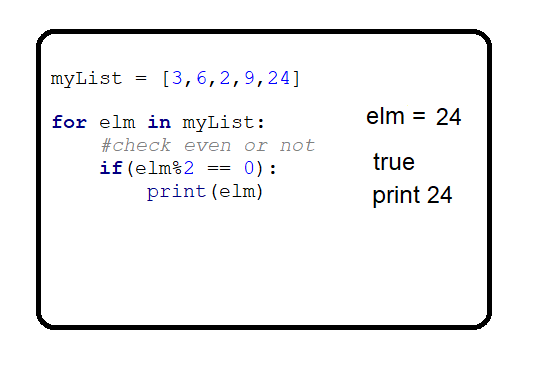Example 2:

For loop on range() function

Find the sum of numbers between 2 given numbers

Code:

## Python Code

``````n1 = 2
n2 = 5
sum = 0
for elm in range(n1, n2+1):
sum += elm

print(sum)
``````

Output: 14

Explanation:  range() function will generate a sequence of numbers between 2 numbers (2nd number not included), so in our case range() generates a sequence of numbers from 2 to 5 i.e [2,3,4,5], and these numbers are added to the sum variable one by one in every iteration.

### For loop with else:

For loop with else is same as For loop but else part is added to it, This else part is executed when the loop runs till the last element without terminating in between the iterations. And the else part is not executed when the loop is terminated in between the iterations with the break keyword.

For loop with else ( Without break)

Code:

## Python Code

``````myList = [1,3,4]
for elm in myList:
print(elm)
else:
print("Else part executed..!")
``````

Output:

1
3
4
Else part executed..!

Explanation: The loop is not terminated by “break”, and all the elements in the list have been processed, So the else part is also executed.

For loop with else ( With break)

Code:

## Python Code

``````myList = [1,3,4]
for elm in myList:
if elm == 3:
break
print(elm)
else:
print("Else part executed..!")
``````

Output: 1

Explanation: Since the loop is terminated by “break”, so the else part will not be executed.